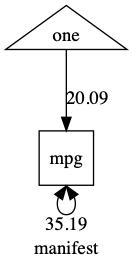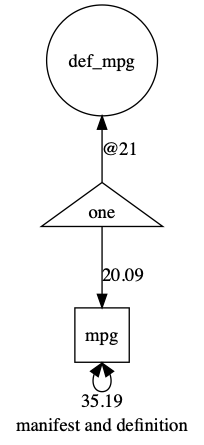# umx!

## Definition variables in umxRAM

We’re used to seeing measured variables as manifests in our models, like this:

``````m1 = umxRAM("my manifest model",data = mtcars,
umxPath(v.m. = "mpg")
)
plot(m1)
``````You can also use definition variables in RAM. A definition variable is a variable that takes a row-specific value in the model.

``````m1 = umxRAM("manifest and definition", data = mtcars,
umxPath(v.m. = "mpg"),
umxPath(defn = "mpg")
)
plot(m1)
``````

Note, this model doesn’t yet serve a useful purpose: It just shows how to add a definition variable to a model.See how this is handled: umx creates a latent variable with variance fixed at zero, and a mean fixed at zero, but sets the label of the mean to data.mpg.

``````umxPath(defn = "mpg")
``````
``````mxPath def_mpg <-> def_mpg [value=0, free=FALSE, lbound=0]

mxPath one -> def_mpg [value=0, free=FALSE, label='data.mpg']
``````

That means the model will use the subject-specific value of mpg in the model. It also means the model will drop all rows where the definition variable is NA.

Note, to avoid confusing the latent variable created by umx with the manifest variable, umx names the latent “def_” so you can refer to the definition variable as ‘def_mpg’

If you want, you can set your own name for the definition variable:

``````m1 = umxRAM("my name my way", data = mtcars,
umxPath(defn = "defMPG", label= "mpg")
)
plot(m1)
``````### To be added to this blog

1. A moderation example: x->y; mod-> y; x*mod->y
2. The same thing using a product column (just a column containing X * Moderator for each row)

moderation and mediation

#Moderation “By Hand”

``````# setwd("location")        # Working directory
set.seed(123)              # Standardizes the numbers generated by rnorm; see Chapter 5
N  = 100                   # Number of participants; graduate students
X  = abs(rnorm(N, 6, 4))   # IV; Hours of sleep
X1 = abs(rnorm(N, 60, 30)) # Adding some systematic variance for our DV
Z  = rnorm(N, 30, 8)       # Moderator; Ounces of coffee consumed
Y  = abs((-0.8*X) * (0.2*Z) - 0.5*X - 0.4*X1 + 10 + rnorm(N, 0, 3)) # DV; Attention Paid
Moddata = data.frame(X, X1, Z, Y)
Moddata\$sleep     = X
Moddata\$coffee    = Z
Moddata\$Attention = Y

summary(Moddata)
``````

# Centering Data

``````Moddata\$Xc = scale(Moddata\$X, center = TRUE, scale = FALSE) # Centering IV; hours of sleep
Moddata\$Zc = scale(Moddata\$Z, center = TRUE, scale = FALSE) # Centering moderator; coffee consumption

Moddata\$sleepC  = as.numeric(scale(Moddata\$X, center = TRUE, scale = FALSE)) # Centering IV; hours of sleep
Moddata\$coffeeC = as.numeric(scale(Moddata\$Z, center = TRUE, scale = FALSE)) # Centering moderator; coffee consumption
Moddata\$sleep_by_coffee = Moddata\$sleepC*Moddata\$coffeeC
``````

# Moderation “By Hand”

``````library(rockchalk)
library(gvlma)
fitMod = lm(Y ~ Xc + Zc + Xc*Zc) # Model interacts IV & moderator

m1 = lm(Attention ~ sleepC + coffeeC + sleepC*coffeeC, data = Moddata) # Model interacts IV & moderator
umxAPA(m1); umxAPA(m1, std=TRUE)

``````

(Intercept) β = 0.03 [-0.07, 0.13], t = 0.67, p = 0.507 sleepC β = 0.75 [ 0.65, 0.85], t = 14.94, p < 0.001 coffeeC β = 0.35 [ 0.26, 0.45], t = 7.11, p < 0.001 sleepC:coffeeC β = 0.26 [ 0.17, 0.35], t = 5.66, p < 0.001

ps = plotSlopes(m1, plotx= “sleepC”, modx= “coffeeC”, xlab= “Sleep”, ylab = “Attention Paid”, modxVals = “std.dev”) visreg(m1, xvar = “sleepC”, by = “coffeeC”)

m1 = umxRAM(“moderate”, data = Moddata, umxPath(c(“sleep_by_coffee”, “sleepC”, “coffeeC”), to = “Attention”), umxPath(unique.bivariate= c(“sleep_by_coffee”, “sleepC”, “coffeeC”)), umxPath(v.m. = c(“sleep_by_coffee”, “sleepC”, “coffeeC”, “Attention”)) )

umxSummary(m1, showEstimates = “std”) plot(m1)

|Name | CI | |:——————————|-:——————-| |sleepC_to_Attention | 0.75 [0.66, 0.85] | |coffeeC_to_Attention | 0.35 [0.25, 0.46] | |sleep_by_coffee_to_Attention | 0.28 [0.18, 0.39] | |Attention_with_Attention | 0.23 [0.15, 0.31] | |coffeeC_with_sleepC | -0.13 [-0.32, 0.06] | |sleep_by_coffee_with_sleepC | 0.16 [-0.03, 0.35] | |coffeeC_with_sleep_by_coffee | -0.03 [-0.23, 0.17] |  “χ²(0) = 0, p = 1.000; CFI = 1; TLI = 1; RMSEA = 0”

# Mediation

set.seed(123) # Standardizes the numbers generated by rnorm; see Chapter 5 N = 100 #Number of participants; graduate students X = rnorm(N, 175, 7) #IV; hours since dawn M = 0.7X + rnorm(N, 0, 5) #Suspected mediator; coffee consumption Y = 0.4M + rnorm(N, 0, 5) #DV; wakefulness Meddata = data.frame(X, M, Y)

library(mediation) ?mediate fitM = lm(M ~ X, data=Meddata) #IV on M; Hours since dawn predicting coffee consumption fitY = lm(Y ~ X + M, data=Meddata) #IV and M on DV; Hours since dawn and coffee predicting wakefulness gvlma(fitM) #data is positively skewed; could log transform (see Chap. 10 on assumptions) gvlma(fitY) fitMed = mediate(fitM, fitY, treat=”X”, mediator=”M”) summary(fitMed) plot(fitMed) fitMedBoot = mediate(fitM, fitY, boot=TRUE, sims=999, treat=”X”, mediator=”M”) summary(fitMedBoot) plot(fitMedBoot)